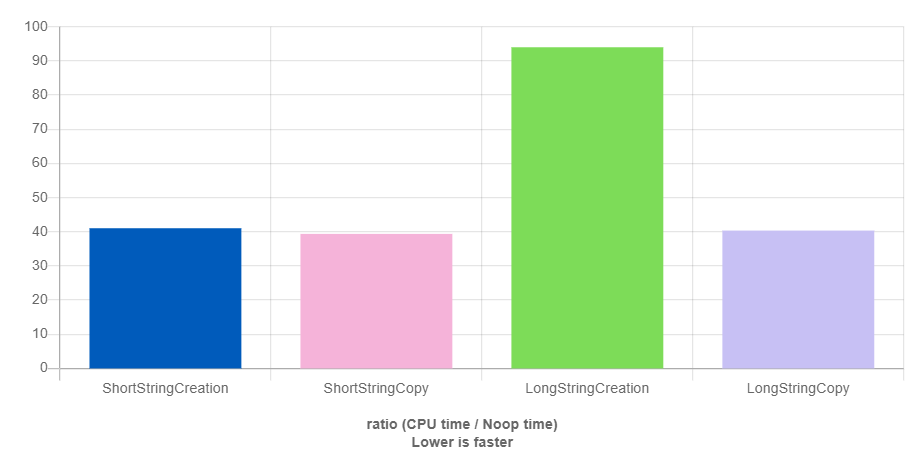C++ 的 std::string 是我們在學 C++ 最早且最常使用的函示庫了，我們在學習的時候大多是去理解 string 就是一個容器（container），基本上大概可以想成 std::vector<char>

struct {
CharT* ptr;
size_type size;
size_type capacity;
};


const char* SHORT_STR = "hello world";

void ShortStringCreation(benchmark::State& state) {
// 不斷重新產生字串
// 因為短字串最佳化的關係，不需要分配新的記憶體
for (auto _ : state) {
std::string created_string(SHORT_STR);
// 必須加上這行來避免編譯器過度優化
benchmark::DoNotOptimize(created_string);
}
}
BENCHMARK(ShortStringCreation);

void ShortStringCopy(benchmark::State& state) {
// 產生字串物件一次，然後不斷重複複製
std::string x; // create once
for (auto _ : state) {
x = SHORT_STR; // copy
benchmark::DoNotOptimize(x);
}
}
BENCHMARK(ShortStringCopy);

const char* LONG_STR = "this will not fit into small string optimization";

void LongStringCreation(benchmark::State& state) {
// 長的字串會觸發記憶體分配的機制
for (auto _ : state) {
std::string created_string(LONG_STR);
benchmark::DoNotOptimize(created_string);
}
}
BENCHMARK(LongStringCreation);

void LongStringCopy(benchmark::State& state) {
// 重新使用記憶體，所以複製的話速度會有提升
std::string x;
for (auto _ : state) {
x = LONG_STR;
benchmark::DoNotOptimize(x);
}
}
BENCHMARK(LongStringCopy);（圖一：使用 GCC + libstdc++ 短字串與長字串生成和複製的效能比較）

struct {
size_type size;
size_type capacity;

// union 取其最大 size 的物件為記憶體 size，這邊為 16
union {
CharT* ptr;
std::array<char, 16> short_string;
}
};


// example1.cc
#include <string>
#include <cstdio>
#include <cstdlib>

std::size_t allocated = 0;

void *operator new(size_t sz)
{
void *p = std::malloc(sz);
allocated += sz;
return p;
}

void operator delete(void *p) noexcept
{
return std::free(p);
}

int main()
{
allocated = 0;
std::string s("***"); // 短字串
std::printf("stack space = %zu, heap space = %zu, capacity = %zu\n",
sizeof(s), allocated, s.capacity());

allocated = 0;
std::string s2(s.capacity() + 1, '*'); // 長字串
std::printf("stack space = %zu, heap space = %zu, capacity = %zu\n",
sizeof(s2), allocated, s2.capacity());

allocated = 0;
s2.push_back('x');
std::printf("stack space = %zu, heap space = %zu, capacity = %zu\n",
sizeof(s2), allocated, s2.capacity());
}


$clang++ -std=c++20 -stdlib=libstdc++ example1.cc; ./a.out stack space = 32, heap space = 0, capacity = 15 stack space = 32, heap space = 17, capacity = 16 stack space = 32, heap space = 33, capacity = 32  第一個字串物件因為是短字串，所以直接使用 string 裡面預留的空間，因此可以看到 heap 使用空間為 0。接著第二個字串存的是一個長字串（比短字串的空間再多一個），所以 string 觸發 new 去分配新的 heap 來儲存字串，可以發現 heap 空間比 capacity 多一個，這是因為實際上最後還會補上一個終止符號（Terminal Null）\0。當我們在長字串後面 push_back 一個字元時，其機制跟 std::vector 一樣，會重新分配兩倍大的 heap 來作為新的預留空間。 同樣的實驗我們換成 Clang + libc++ 跑跑看： $ clang++ -std=c++20 -stdlib=libc++ example1.cc; ./a.out
stack space = 24, heap space = 0, capacity = 22
stack space = 24, heap space = 32, capacity = 31
stack space = 24, heap space = 0, capacity = 31